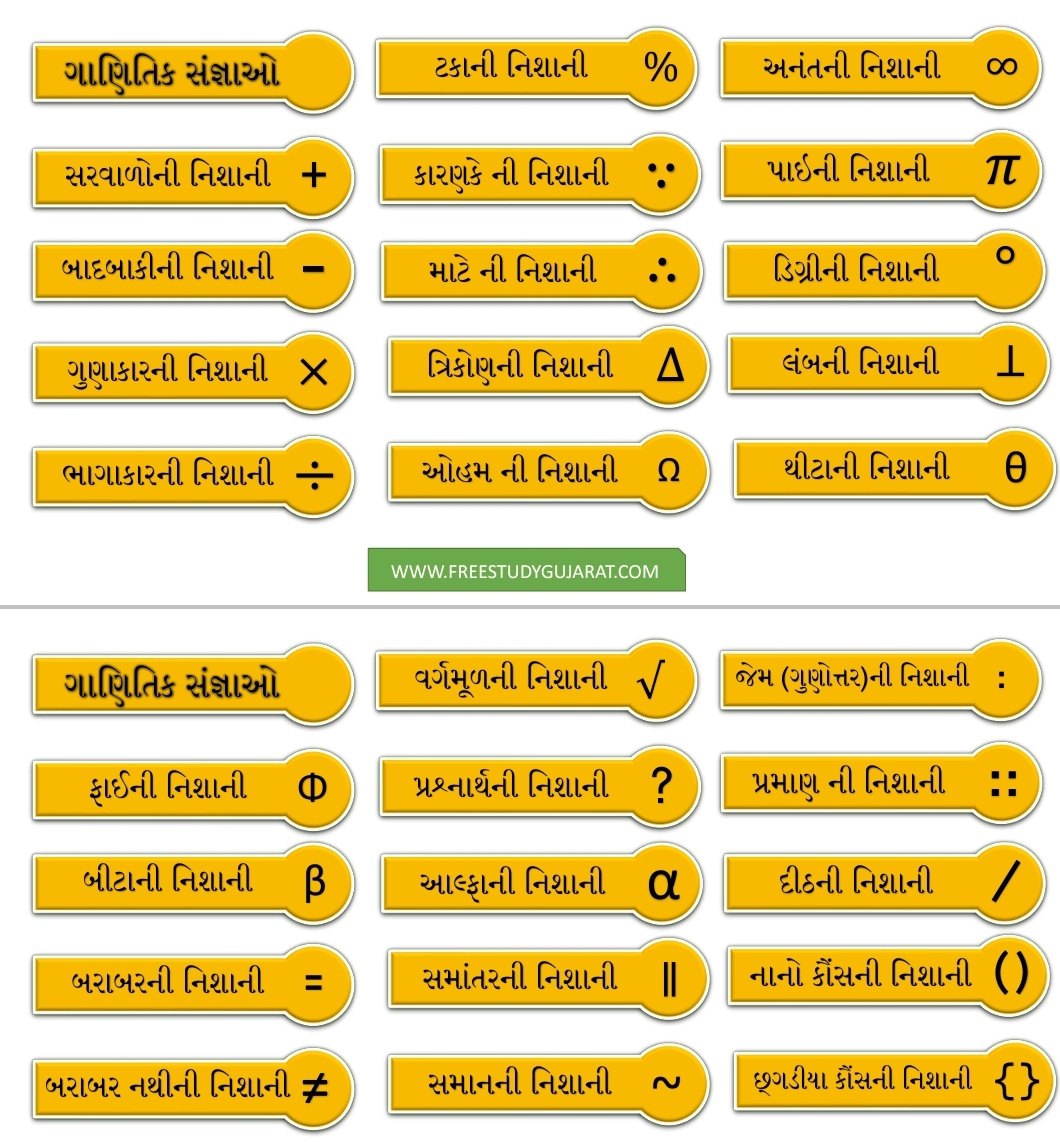## Thursday, April 22, 2021

### MATHS USEFUL SUTRO ANE SANGNAO NI USEFUL PDF FILE.

MATHS USEFUL SUTRO ANE SANGNAO NI USEFUL PDF FILE.

NCERT Maths Formula Gujarati In Gujarati for STD 6 to 8 students. It is app about Maths formula in Gujarati Language with new course

A mathematical symbol is a figure or a combination of figures that is used to represent a mathematical object, an action on mathematical objects, a relation between mathematical objects, or for structuring the other symbols that occur in a formula. As formulas are entierely constitued with symbols of various types, many symbols are needed for expressing all mathematics.

The most basic symbols are the decimal digits (0, 1, 2, 3, 4, 5, 6, 7, 8, 9), and the letters of the Latin alphabet. The decimal digits are used for representing numbers through the Hindu–Arabic numeral system. Historically, upper-case letters were used for representing points in geometry, and lower-case letters were used for variables and constants. Letters are used for representing many other sort of mathematical objects. As the number of these sorts has dramatically increased in modern mathematics, the Greek alphabet and some Hebrew letters are also used. In mathematical formulas, the standard typeface is italic type for Latin letters and lower-case Greek letters, and upright type for upper case Greek letters

- All NCERT new course Std 6 to 8 Maths Formula covered in the app.

- New NCERT syllabus added in this version.

- Small size of just 3 MB.

- Easy & Simple UI and Design.

- Easy to use.

- Useful for Students of Std 6-7-8 & their teachers.

- Useful for competetive exams like TET, TAT, GPSC, BANK & more.Useful PDF File For All The Students, Teachers And Competitive Examination Aspirnat Students of Gujarat.

USEFUL FILE CREATED BY

K M SHAH

ANAND